# Adapted reference input to control PID-based active suspension system

Adapted reference input to control PID-based active suspension system

Adel DjellalRabah Lakel

Department of Second Cycle School of Engineer in Industrial Technologies-Annaba Cité Safsaf, Annaba, Algeria

Department of Electronics Badji Mokhtar University - Annaba Annaba, Algeria

Corresponding Author Email:
a.djellal@epst-annaba.dz
Page:
7-23
|
DOI:
https://doi.org/10.3166/JESA.51.7-23
Received:
|
Accepted:
|
Published:
30 June 2018
| Citation

OPEN ACCESS

Abstract:

In this paper, active suspension system is controlled using PID controller with adapted reference point. After the derivation of quarter car suspension model. Three approaches were applied: passive suspension system, active suspension system with constant reference and with adapted reference. The proposed approach was focusing on system life span; how to reduce brutal controller actions, that can cause car body damage, and ensure a certain ride comfort? Simulation of three approaches has been done using quarter car system and MATLAB simulation model to implement the proposed technique and compare performance variation in different cases: road bump and other road disturbances. Through this study, it was found that the proposed active suspension model may serve as an interesting benchmark for several profound studies on filter model and controller design.

Keywords:

active suspension system, PID controller, quarter car model, passive suspension system

1. Introduction

Conventionally, automotive suspension designs have to compromise between three criteria; road holding, load carrying and passenger comfort. Usually, the suspension must support the vehicle, improve handling while steering, and assure a certain isolation of passengers and loads from road disturbances. Therefore, Good ride needs a soft suspension whereas applied load requires stiff suspension. According to ISO 2631-1 standard, exposing the human body to vibrations with frequencies between 0.5-80 Hz could probably cause a great risk of injury to the vertebrae in the lumber region and the nerves connected to these segments (Chamseddine et al., 2006).

Due to these conflicting demands, suspension design had to be determined by the type of use for which the vehicle was designed. However, Active suspensions are considered a way to auto adapt suspension stiffness according to the road needs. Therefore, Active suspension is a very fertile topic with new innovations each year.

In 1991, (Sunwoo, 1991) proposed a model reference adaptive control (MRAC) technique for vehicle active suspension system. In 1995, (Alleyne and Hedrick, 1995) worked on active suspension control using nonlinear adaptive control. In 2004, (Kruczek and Stribrsky, 2004) and (Rossi and Lucente, 2003) used

control design; the first worked on Active Suspension with linear motor, and the second worked on semi-active suspension. In 2006, (Sam and Hudha, 2006) and (Chamseddine et al., 2006) worked on Active Suspension control and design, the first worked on modelling and force tracking control of hydraulic actuator, and the second worked on sliding mode techniques. In 2009, (Eski and Yildirim, 2009) worked on vibration control of vehicle active suspension system using a new robust neural network control system. And (Hyniova et al., 2009) worked on optimizing energy efficiency of linear electric motor based active suspension system. In 2011, (Conde and Carbajal, 2011) used Generalized Proportional Integral (or GPI) design to control quarter-car active suspension system. In 2012, (Choudhury and Sarkar, 2012) compared Passive Suspension with PID controller based Active Suspension system. In 2013, (Li et al., 2013) used adaptive sliding-mode control problem for nonlinear active suspension systems via the Takagi-Sugeno (T-S) fuzzy approach. Finally, in 2017, (Jamil et al., 2017) presented a comparison between passive, proportional-integral-differential (PID) and multi-order PID (MOPID) controlled active suspensions.

Two common assumptions are taken into account in all these works; constant reference for all control techniques, and vehicle representation using so-called ’quarter car’ model (Crolla, 1996). In this work, adaptive reference is proposed to reduce brutal controller actions that can cause damage to the vehicle system.

The remainder of this paper is organized as follows: Section 2 introduces mathematical model of vehicle, disturbance, etc. Section 2 presents the PID controller used to control suspension. Section 3 describes model simulation and comparison between passive suspension and active suspension with constant and adapted reference. Section 4 analyzes simulation results. Finally, Section 5 concludes the work with general study of performances of different techniques, and future works.

2. Mathematical modeling

In this part, a full model of the suspension dynamics and road disturbances is described. This shall describe the full model for a rigorous computer simulation study to be carried out. The mathematical model for suspension system is given using the Newtonian mechanics, the suspension system is model based on a quarter car configuration.

The active suspension system is designed and model using the feedback control element embedded into the system. For simplification purposes, the active system is modeled as an action applied directly to the chassis and the tire.

2.1. Quarter car

Quarter car model (Wilson et al., 1986) is frequently used for suspension system analysis and design due to its simplicity and yet ability to present many important parameters. Extension to one-half car model has been described in (Rossi and Lucente, 2003) and full car model in (Eski and Yildirim, 2009).

Figure 1a presents Quarter passive car suspension system. Car body is denoted as sprung mass and the tire is denoted un-sprung mass. Single wheel axle is connected to the quarter portion of the car body through a passive spring and damper. The tire is assumed to have only the spring constant and is in permanent contact with the road at the other end. The road serves as an external disturbance to the system.

Based on Newtonian mechanics, the equations of the motion for the passive suspension system are given as (Mailah and Priyandoko, 2005):

$m_{S} \frac{d^{2} z_{S}}{d t^{2}}=-k_{S}\left(Z_{S}-Z_{u}\right)-C_{S}\left(\frac{d z_{S}}{d t}-\frac{d z_{u}}{d t}\right)$        (1)

$m_{u} \frac{d^{2} z u}{d t^{2}}=-k_{s}\left(z_{s}-z_{u}\right)+k_{t}\left(z_{u}-z_{r}\right)+C_{s}\left(\frac{d z_{s}}{d t}-\frac{d z_{u}}{d t}\right)$        (2)

Where:

$\boldsymbol{Z}_{\boldsymbol{r}}$         Road displacement

$\boldsymbol{Z}_{\boldsymbol{s}}$         Car body displacement

$\mathbf{z}_{\boldsymbol{u}}$         Un-sprung mass displacement

$\boldsymbol{C}_{\boldsymbol{s}}$         Damping coefficient

$\boldsymbol{m}_{\boldsymbol{s}}$       Sprung mass

$\boldsymbol{m}_{\boldsymbol{u}}$       Un-sprung mass

$\boldsymbol{k}_{\boldsymbol{s}}$         Spring stiffness constant

$\boldsymbol{k}_{t}$         Tire stiffness constant

Quarter car model for active suspension system can be obtained by adding an actuator parallel to spring and damper. Figure 1b shows a schematic of a quarter car model for active suspension system.

The equations of motion for active suspension system are given as (Mailah and Priyandoko, 2005):

$m_{S} \frac{d^{2} z_{S}}{d t^{2}}=-k_{S}\left(z_{S}-z_{u}\right)-C_{S}\left(\frac{d z_{S}}{d t}-\frac{d z_{u}}{d t}\right)+f_{a}$           (3)

$m_{u} \frac{d^{2} z_{u}}{d t^{2}}=-k_{s}\left(z_{s}-z_{u}\right)+k_{t}\left(z_{u}-z_{r}\right)+C_{s}\left(\frac{d z_{s}}{d t}-\frac{d z_{u}}{d t}\right)-f_{a}$        (4)

Where, $f_{a}$  is the actuator force.

## 1.png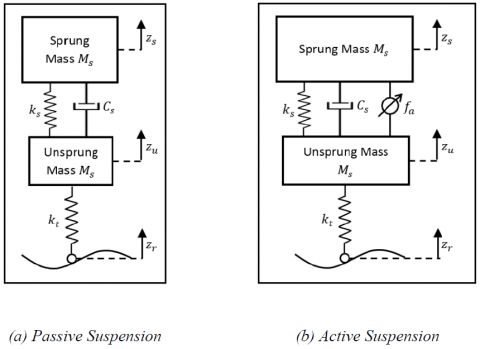Figure 1. Quarter car system

2.2. Disturbance model

A road bump will be used as a disturbance to the vehicle system in this work. The bump was assumed as a sinusoidal form. Some works (Sam and Hudha, 2006; Conde and Carbajal, 2011) used a road disturbance in function of time, this type of bump does not take into account the vehicle speed. However, in our work, we will study the case of different car speeds.

The following figure illustrates the dimension of the disturbance model according to (Choudhury and Sarkar, 2012):

## 2.jpgFigure 2. Disturbance model

2.3. Filter model

For standard passive suspension systems, if the comfort is improved, then handling stability is deteriorated. This problem is the main reason why active suspension systems are used. However, most active suspension systems (Choudhury and Sarkar, 2012; Conde and Carbajal, 2011; Sunwoo, 1991) aim to bring car body into equilibrium value, which always needs high energy, or in some cases, can cause damage to the tire and/or suspension system. Therefore, in this work, the active suspension tries to bring car body to comfortable situation according to tire movements in such way that annoying frequencies must be eliminated (according to (Kruczek and Stribrsky, 2004), they can be ranged between 4 and 8 Hz).

## 3.jpgFigure 3. Proposed Active suspension system

The filter was chosen in such way to ensure a comfortable ride and taking into account sudden displacements. The figure bellow shows the step response of the proposed filter. For a step disturbance, the desired displacement must stabilize after about 1 second so the passengers will feel a minor annoyance.

The filter transfer function is as follows:

$F(s)=\frac{50}{s^{2}+15 s+50}$            (5)

2.4. Model parameters

Modeling parameters are taken for a sedan vehicle. The parameters used in the study are taken from (Conde and Carbajal, 2011):

Table 1. Vehicle parameters

 Parameter name Parameter symbol Value Sprung mass (kg) $m_{s}$ 282 kg Unsprung mass (kg) $m_{u}$ 45 kg Tire stiffness constant (N/m) $k_{t}$ 165790 N/m Spring stiffness (N/m) $k_{s}$ 17900 N/m Damping constant (N.s/m) $C_{t}$ 1000 N.s/m

With these parameters and the given cinematic equations, and with assuming that the initial values are zero. The system can be written as follows:

$Z_{S}(S)=\frac{1000 s+17900}{282 s^{2}+1000 s+17900} Z_{u}(s)+\frac{1}{282 s^{2}+1000 s+17900} f_{a}(s)$         (6)

$z_{u}(s)=\frac{165790}{45 s^{2}+1000 s+183690} z_{r}(s)+\frac{1000 s+17900}{45 s^{2}+1000 s+183690} z_{s}(s)$

$-\frac{1}{45 s^{2}+1000 s+183690} f(s)$          (7)

3. Instrumentation of active suspension system

3.1. Measurements required

As in (Conde and Carbajal, 2011), the only required variable for implementation of the proposed controller are the vertical displacement of the body of the car $z_u$ , and the vertical displacement of the wheel $z_s$ . These variables are needed to control actuator according to measured values.

3.2. Sensors

In (Chamseddine et al., 2006), the use of sensors in experimental and commercial vehicles is detailed. The most common sensors used for measuring the vertical displacement of the body and the wheels, are laser sensors (Conde and Carbajal, 2011). Accelerometers and other sensors that measure $z_u$  and $z_s$ are not needed since it is possible to integral reconstruct them from the knowledge of body and wheel displacements.

The schematic diagram of the instrumentation of active suspension system is illustrated as follows:

## 4.png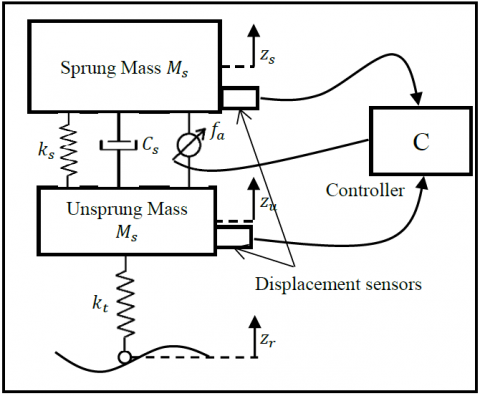Figure 4. Schematic diagram of the instrumentation of the active suspension system

3.3. Linear electric motor

## 5.jpgFigure 5. GSM series electric linear actuator by EXLAR

In (Hyniova et al., 2009), Linear electric motor has been used as an actuator to generate needed forces. Figure 5 shows an example of a commercialized linear motor (GSM Series by Exlar). The main interest of linear motors is that they directly translate electrical energy into usable linear mechanical force and motion.

Linear motor movements take place with high velocities (more than 1000 mm/s), large accelerations (up to g multiples), and forces (up to 10 kN). However, there is an important question whether the linear motor model could be omitted or not? The answer is ’yes’ (Hyniova et al., 2009). Both electrical and mechanical constants are small (just above 1 ms).

4. PID controller design

PID controller has been widely used in industry because of its simplicity and effectiveness. Despite many uses of the PID controller, its standard structure has constant gain parameters and is not good to decrease velocity control error (Eski and Yildirim, 2009). Therefore, exponential function is added to derivate component of conventional PID controller.

The used PID controller was adapted from (Eski and Yildirim, 2009) and can be described as follows:

$f_{a}(t)=G \cdot\left(K_{p} e(t)+K_{i} \int e(t) d t+K_{d} \frac{d e(t)}{d t}\left(K_{N} e^{-K_{N} t}\right)\right)$           (8)

Where $K_{p}, K_{i}, K_{d}$ and $K_{N}$ are the PID controller gains.

Random tuning technique has been used to optimize controller parameters using step response that will be analyzed later. The Fitness function, that has to be minimized, is defined as follows:

$J=\frac{1}{T} \int_{0}^{T}\left(z_{s}-z_{f}\right)^{2} d t \times \frac{1}{T} \int_{0}^{T}\left(z_{c}-z_{u}\right)^{2} d t$          (9)

With $Z_{f}$ is filter output for Active Suspension with adapted reference. For the constant reference, $Z_{f}$

is assumed to be zero. And T is the simulation period, which in our case equals to 5 seconds. Method of trial and error is used to determine the parameters of PID controller.

After 5000 iterations, the parameters for adapted reference (left), and constant reference (right) were set to:

\left\{\begin{aligned} G &=15000 \\ K_{p} &=4.9336 \\ K_{i} &=4.7032 \\ K_{d} &=0.4736 \\ K_{N} &=283.5086 \end{aligned}\right.           (10)

$\left\{\begin{array}{c}{G=15000} \\ {K_{p}=4.9751} \\ {K_{i}=4.9489} \\ {K_{d}=0.3614} \\ {K_{N}=414.1968}\end{array}\right.$            (11)

5. Model simulation

To validate the proposed control technique, the mathematical model is transformed to computer simulation model. As in (Choudhury and Sarkar, 2012), model variable-step continuous solver (ODE45 or Dormand-Prince) is used which is based on an explicit Runge-Kutta formula. It is a one-step solver; so, to compute $y\left(t_{n}\right)$

, it needs only the solution in the immediately preceding time point $y\left(t_{n-1}\right)$ .

5.1. Passive suspension system

The passive suspension system was modeled in Simulink as shown in Figure 6. this model was built using the equations 1 and 2 by supposing that there is no external actuator ($\boldsymbol{f}_{\boldsymbol{a}}=\boldsymbol{0}$). The model has one input (

$\mathbf{Z}_{\boldsymbol{r}}$) and two outputs ($z_{s}$ and $z_{u}$).

## 6.jpg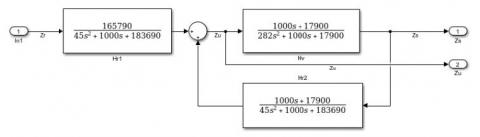Figure 6. Passive Suspension system simulation model

5.2. Active suspension system with constant reference

Active suspension system requires actuator force to provide external control, the goal of this actuator to provide a better ride and handling. The force fa is given by a PID controller that aims to bring the body position (

$z_{s}$) to constant value (zero). The Simulink model was built based on equations 3 and 4. The system has one input ( $z_{r}$) and three outputs ( $z_{s}, z_{u}$ and $f_{a}$ ).

## 7.jpgFigure 7. Active suspension system with constant reference

5.3. Active suspension system with adapted reference

As it is seen, the previous Active suspension system tries to bring the car body to the position that ensures comfortable ride without taking into account sudden road disturbances that can cause damage to the wheel and/or suspension system. The following configuration, the actuator tries to bring car body to a comfortable ride in accordance with wheel displacements.

## 8.jpgFigure 8. Active suspension system with adapted reference

6. Simulation results

In the simulation, two cases were taken into account: different car speed and different road disturbances. Comparisons of the car body displacement and needed force for different case are discussed.

6.1. Different car speed

For this case, road disturbance presented in Figure 2 is taken as reference and car speed will by varied for the three suspension systems. Figure 9 shows the displacement of sprung mass for different speed to show the speed influence into car displacement and Figure 10 shows actuator force for different speeds.

## 9.pngFigure 9. Body displacement for different car speeds

From results, it can be seen that passive Suspension System suffers from high displacement rates (up to 20 cm) for low speeds. Though, the classical active suspension system reduced disturbance to 3 cm, and the proposed system gave a displacement up to 5 cm. However, if the car speed is increased, the body displacement for the three systems are similar. Moreover, the proposed system needed less force for small speeds. This is due to the control system that tries to reduce disturbance with taking into account sudden vibrations.

## 10.pngFigure 10. Actuator force for different car speeds

To have a more elaborate idea on the tested techniques, the following table contains a comparison between different results:

Table 2. Displacement, force and wheel deformation for different speeds

 Speed (km/h) Maximum displacement speed (m/s) Maximum Force (N) Wheel deformation (cm) Passive Active 1 Active 2 Passive Active 1 Active 2 Passive Active 1 Active 2 5 1.2035 0.2534 0.3857 / 3002.1 1798.9 2.06 2.04 1.94 15 0.9409 0.5924 0.5797 / 4695.2 3677.5 5.42 6.20 5.79 25 0.9531 0.7153 0.6473 / 4964.5 4219.2 11.53 14.59 14.28 35 0.8652 0.6396 0.6198 / 4572.9 4096.9 17.19 19.27 19.25 45 0.7547 0.5640 0.5609 / 4017.7 3736.3 16.70 17.80 17.84 55 0.6546 0.5014 0.4970 / 3497.9 3327.9 14.72 15.55 15.59

Table 2 is comparison between studied systems for different car speeds from 5 km/h to 55 km/h. the studied systems are passive suspension (Passive), Active Suspension with constant reference (Active 1) and Active Suspension with adapted reference (Active 2). the presented parameters are:

• Maximum displacement speed ( $m / s$ ), which is the maximum vertical body speed;
• Maximum force ( $N$ ), which is the maximum value of exerted force during bump passing;
• Wheel deformation ( $\boldsymbol{c m}$ ), which is the difference between wheel zu and road zr position or

$\boldsymbol{w}_{\boldsymbol{d}}=\left|\mathbf{z}_{\boldsymbol{u}}-\mathbf{z}_{\boldsymbol{u}}\right|$

## 11.pngFigure 11. Maximum displacement speed and force for different car speeds

Two aspects can be retrieved from Table 2:

1. Car speed influence on system behavior is very important; wheel deformation is very important from about 20 km/h, body displacement speed is stable between 15 and 30 km/h and the maximal exerted force is at its highest value between 20 and 30 km/h. Therefor, 15 km/h is the best car speed to pass through road bump;
2. For car speeds between 5 and 20 km/h, the proposed approach needed less force (up to 40% less), but body displacement speed was greater than classic Active Suspension. However, between 20 and 40 km/h, body displacement speed and maximal exerted force for proposed approach were the lowest. for car speeds more than 40 km/h, systems gave more or less very near performances.

To have more approximate view of body speed influence on systems, Figure 11 shows maximum displacement speed and maximum exerted force for different car speeds.

6.2. Different road disturbances

In this case, the systems were tested for different road types: random disturbance, road bumps, step, ramp, etc. this comparison is used to study control robustness for different inputs.

6.2.1. Random disturbance

The first case is random disturbance with given maximum acceleration ( $\ddot{z}_{r m a x}=100^{m} / s^{2} \approx 10 G$ ). It can be seen (from Figure 12) that passive suspension system moves with high velocity (up to 0.591 m/s). While active suspension with constant reference moves with low speed (0.139 m/s vs. 0.254 m/s for our approach). However, if we observe the force efficiency (Figure 13), active suspension with adapted reference needed less force (30% less).

## 12.png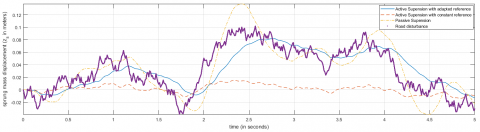Figure 12. Random disturbance ( $\ddot{\mathbf{z}}_{r m a x} \approx 10 G$ )

## 13.jpg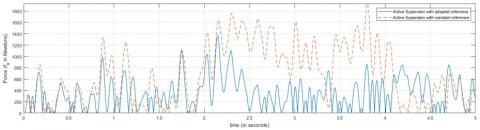Figure 13. Actuator force for random disturbance ( $\ddot{\mathbf{z}}_{r m a x} \approx 10 G$ )

6.2.2. Step input

The second test was made using step input (final value = 10 cm). In this case, the disturbance is a sudden displacement of 10 cm, it can be seen that passive suspension has large displacement speed (up to 0.712 m/s) and overshoot (up to 16 cm) which causes ride inconvenience. However, active suspension with constant reference tries to stay at zero reference, which needs a lot of energy (see exerted force in Figure 15). Moreover, the proposed approach has less displacement speed (up to 0.481 m/s vs 0.478 m/s for classic Active Suspension) and needed less maximal force (up to 15% less).

## 14.pngFigure 14. Step response (final value = 10 cm)

## 15.pngFigure 15. Actuator force for step respond (final value = 10 cm)

7. Conclusion

This study intended to present a new approach to control active suspension system of a quarter car system. Simulation results shows that the technique performs better than passive suspension system for different disturbances. However active suspension system with constant reference gave better results compared to the proposed technique, this is due to the controller tending to bring the body displacement to constant value which is the best comfort option. Though, this last technique does not take into account brutal road displacements that can cause system damage. Therefore, the proposed technique tries to bring the car body to a certain comfort which takes into account sudden road displacement in such way to assure a certain ride comfort. Although, the study has some limitations:

-Improving PID controller design and use other controllers (e.g. Fractional Order PID (Jamil et al., 2017), or Fuzzy PID (Du et al., 2017)) to improve system results.

-Try adaptive control techniques for the case where system parameter changes like body weight.

-Improve filter design so it can be adapted for different road profiles.

-Quarter car suspension give a simple representation of car suspension. However, a more elaborate model would be closer to the real car suspension.

Experimental work is also needed to evaluate the proposed technique with real system parameters.

References

Alleyne A., Hedrick K. J. (1995). Nonlinear adaptive control of active suspensions. IEEE Transactions on Control Systems Technology, Vol. 3, No. 1, pp. 94-101. http://doi.org/10.1109/87.370714

Chamseddine A., Noura H., Raharijaona T. (2006). Control of linear full vehicle active suspension system using sliding mode techniques. In IEEE International Conference on Control Applications. http://doi.org/10.1109/CACSD-CCA-ISIC.2006.4776831

Choudhury S. F., Sarkar R. M. A. (2012). An approach on performance comparison between automotive passive suspension and active suspension system (PID controller) using MATLAB/Simulink. Journal of Theoretical and Applied Information Technology, Vol. 43, No. 2, pp. 295-300.

Conde E. C., Carbajal F. B. (2011). Generalized PI control of active vehicle suspension systems with MATLAB. Applications of MATLAB in Science and Engineering, pp. 335-352. http://doi.org/10.5772/23746

Crolla D. A. (1996). Vehicle dynamics: theory into practice. In Proceedings of the Institution of Mechanical Engineers, Part D: Journal of Automobile Engineering, Vol. 210, No. 2, pp. 83-94. http://doi.org/10.1243/PIME_PROC_1996_210_250_02

Du H., Xiong W., Xu C., Jiang Z. (2017). Research on the controllability and energy saving of the pneumatic direct drive system. International Journal of Heat and Technology, Vol. 35, No. 4, pp. 997-1004. http://doi.org/10.18280/ijht.350436

Eski I., Yildirim S. (2009). Vibration control of vehicle active suspension system using a new robust neural network control system. Simulation Modelling Practice and Theory. Vol. 17, No. 5, p. 778-793. http://doi.org/10.1016/j.simpat.2009.01.004

Hyniova K., Stribrsky A., Honcu J., Kruczek A. (2009). Active suspension system – energy control. IFAC Proceedings Volumes, Vol. 42, No. 19, pp. 146-152. http://doi.org/10.3182/20090921-3-TR-3005.00027

Jamil F., Abdullah M., Ridzuan M., Ibrahim M., Ahmad F. (2017). Multi-order PID control for a simple suspension system. In Proceedings of Innovative Research and Industrial Dialogue’16, pp. 143-144

Kruczek A., Stribrsky A. (2004).  control of automotive active suspension with linear motor. IFAC Proceedings Volumes, Vol. 37, No. 14, p. 365-370. http://doi.org/10.1016/S1474-6670(17)31131-X

Li H., Yu J., Hilton C., Liu H. (2013). Adaptive sliding-mode control for nonlinear active suspension vehicle systems using T-S fuzzy approach. IEEE Transactions on Industrial Electronics, Vol. 60, No. 8, pp. 3328-3338. http://doi.org/10.1109/TIE.2012.2202354

Mailah M., Priyandoko G. (2005). Simulation of a suspension system with adaptive fuzzy active force control. International Journal of Simulation Modeling, Vol. 6, No. 1, pp. 25-36. http://doi.org/10.2507/IJSIMM06(1)3.079

Rossi C., Lucente G. (2003).  control of automotive semi-active suspensions. IFAC Symposium on Advances in Automotive Control, Vol. 37, No. 22, pp. 559-564. http://doi.org/10.1016/S1474-6670(17)30402-0

Sam Y. M., Hudha K. (2006). Modelling and force tracking control of hydraulic actuator for an active suspension system. 2006 1ST IEEE Conference on Industrial Electronics and Applications. http://doi.org/10.1109/ICIEA.2006.257242

Sunwoo M. (1991). Model reference adaptive control for vehicle active suspension systems. IEEE Transactions on Industrial Electronics. Vol. 38, No. 3, pp. 217-222. http://doi.org/10.1109/41.87590

Wilson D. A., Sharp R. S., Hassan S. A. (1986). The application of linear optimal control theory to the design of active automotive suspension. Vehicle System Dynamics: International Journal of Vehicle Mechanics and Mobility, Vol. 15, No. 2, pp. 105-118. http://doi.org/10.1080/00423118608968846